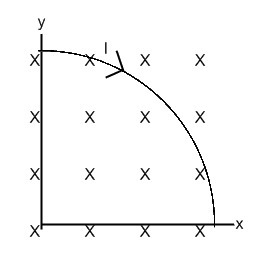# Lorentz force on current-carrying wire

• quasarpulse

## Homework Statement

Given: a quarter-circle of wire (radius r=0.75m) in a uniform 1.7T magnetic field carrying a current of 3.5A (see diagram)Find: The force on the wire.

Note: the connecting wires delivering the current to the quarter circle are parallel to the magnetic field and experience no force.

dF = I dS x B

## The Attempt at a Solution

I know of two ways to solve this.

The first is to use the fact that the force is path-independent and use the F = I L x B formulation; we're not supposed to do that, as this is supposed to be a calculus problem.

The second is the way my instructor suggested to the class, which is to argue by symmetry that the force is directed at a 45 degree angle outward from the origin. I can then treat it like a scalar integration problem and use the fact that the magnitude of dS is r d(theta). It's really a quite simple problem this way, but it only works because the problem is particularly simple.

Having done vector calculus, I feel like there ought to be a third way to attack the thing; it should be possible to parameterize the curve and do something like a line integral. The advantage would be that the same approach would work for a messier problem, where e.g. the magnetic field was nonuniform so the direction wasn't plainly obvious. But I'm not quite sure where to begin. I've got the following parameterization:
x(t) = r sin t
y(t) = r cos t
z(t)=0
0 <= t <= pi/2
but I'm not sure where to go from there. Any ideas?